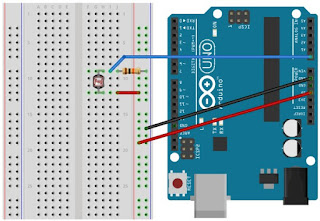# Light Sensor Networks Program and Basic LDR

When you want to make LDR as sensors, then we can refer to the circuit as a voltage divider resistor (see Figure below). By combining the LDR with a resistor (or potentiometer), then we can get the voltage variation (at V1 or V2), which will be input to the Arduino analog pin.### A voltage divider circuit

The voltage at V1 or V2 can be calculated based on ohms law and the rules on the series circuit. In the circuit, the current at all points in the chain of equal value so that we can compute V1 or V2 without knowing the current flowing. Then how to calculate V1 and V2?

In the series, there are three points that have different voltages. Voltage Vin, the voltage at R1, and the voltage on R2. By law ohm, Vin, V1 and V2 can be calculated by:

If you want to calculate V1, then we just substituting between the press 1 and press 2.

Or more commonly known by the formula:

Then if you want to calculate V2, then the formula is:

Based on the workings of the circuit, the circuit for the light sensor is as follows:#### Light sensor and Arduino

The series is based on the above, you need to prepare is a 10K ohm resistor, LDR, and some jumper cables. In order to not try, please resistor 10K ohm potentiometer you can replace with 50 K or 100 K, making it easier when trying with different resistance. Potentiometers can also be used to calibrate the input to the Arduino.

1. One leg LDR is connected to VCC on Arduino
2. One leg resistor connected to GND on arduino
3. Connect the foot rest and foot rest LDR resistor, then the connection is connected to the A0 pin on the Arduino board

## Light Sensor Program

```// Free Tutorial Arduino
// www.ioisalman.com

// A0 pin to LDR
const int pinLDR = A0;

void setup () {
Serial.begin (9600);
pinMode (pinLDR, INPUT);
}

dataLDR int = 0;
void loop () {
Serial.print ("dataLDR:");
Serial.print (dataLDR);
Serial.print ("Conditions");
if (dataLDR <150) {
Serial.println ("DARK");
} Else if (dataLDR <300) {
Serial.println ("dimmed");
} Else if (dataLDR <450) {
Serial.println ("Bright");
} Else {
Serial.println ("glare");
}

delay (1000);
}<150 dataldr="" delay="" dimmed="" else="" glare="" if="" pre="" right="" serial.println="">

```
Program on above will read the value of the voltage on the sensor and sends it to a computer via serial communication. With Arduino, we can make a variety of logic to the light sensor so that the application of LDR can be expanded and made more complex integrated with various systems.

1.